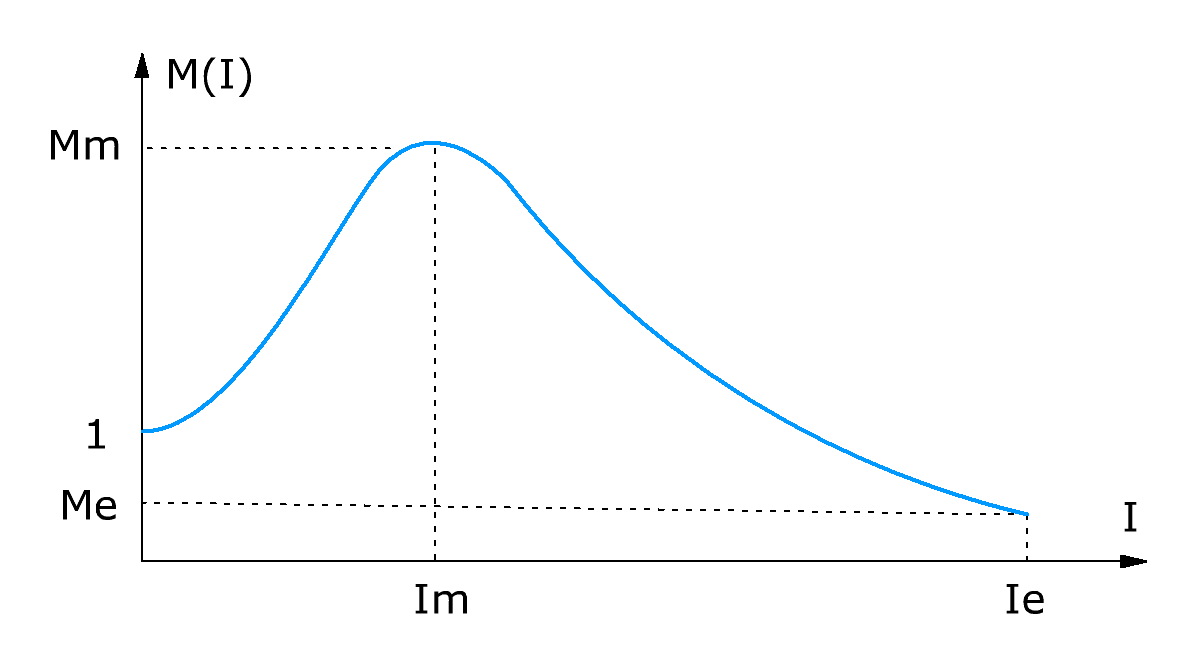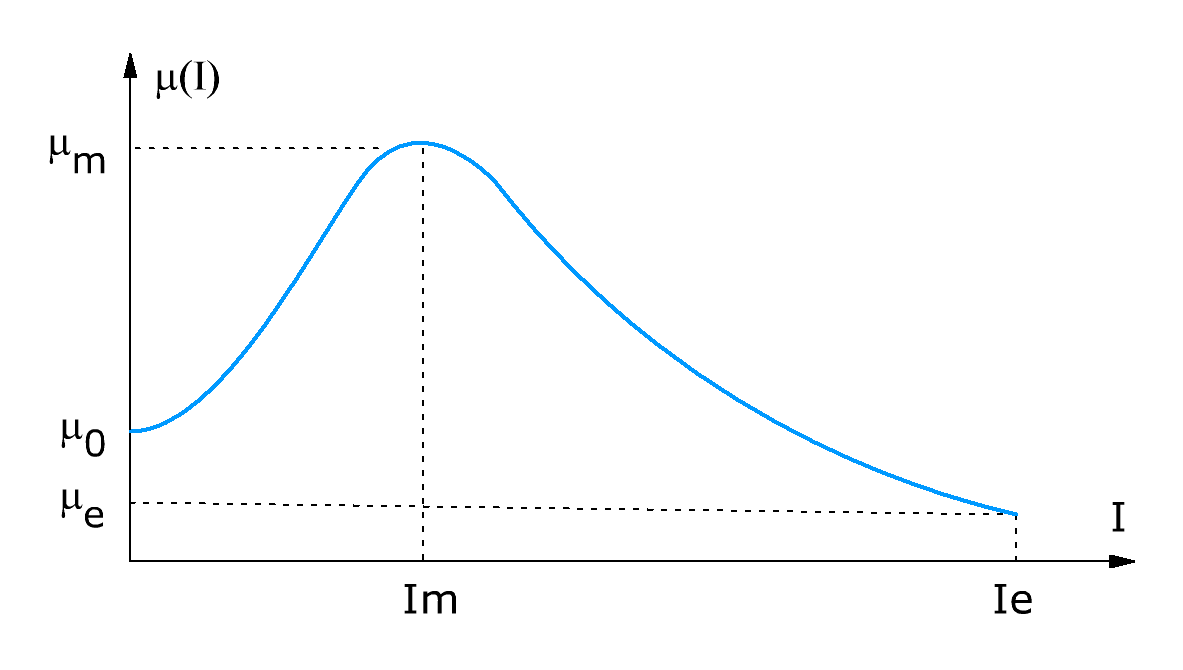Research website of Vyacheslav Gorchilin
2017-09-23
Stoletov curve approximation for ferromagnets
Approximation itself is some kind of approximation to a real function, which allows it to be investigated analytically, and even to "look" into it. beyond the data provided . In this appendix, we will show a rather successful approximation of the Stoletov curve , which allows one to analytically study the dependence of the magnetic permeability on the magnetic field strength $$\mu(H)$$ for ferromagnets, for which $$\mu(H) \gg 1$$. This will require only three points from the measurement graph. If it is necessary to investigate the dependence of the relative inductance on the current $$M(I)$$, then only two such points are needed. We will approximate it, and we will show a more general approach a little lower.
The following approximation for the curve Stoletov: $M(I) = A \exp\left(\frac{-I}{I_0}\right) + \left(B - A \exp\left(\frac{-I}{I_0}\right)\right) \sin\left(\frac{\pi I}{I+I_0}\right) \qquad (1)$ unfortunately, the accuracy of its approximiately poor for calculations which requires minimum deviation from the real curve, it is not applicable. For a more accurate approximation you can use the standard approach, but it will require a power series with 6-7 members, the search of coefficients which will be quite complicated task: $M(I) = 1 + k_1\,I + k_2\,I^2 + k_3\,I^3 + k_4\,I^4 + k_5\,I^5 + \ldots \qquad (2)$ And even then the accuracy of the approximation will remain low not to mention the extrapolation abilities of the approximating function to predict the behavior of a real function outside of the measurement points.
Based on the studies of real dependences $$\mu(H)$$, the author has developed a more simple approximation function, allows you to fairly accurately closer to the real curve and even predict its behavior outside measurements.This will require only two points of the investigated graph — ($$M_m, I_m$$) and ($$M_e, I_e$$), and finding the coefficients of the polynomial will not be too difficult.
In the beginning the author had based the following polynomial: $M(I) = {1 + k_{11} I + k_{12} I^2 + k_{13} I^3 \over 1 + k_{21} I + k_{22} I^2 + k_{23} I^3} \qquad (3)$ But as a result of experiments proved that it is possible to remove some of the members maintaining the approximation accuracy (for the curve Stoletov): $M(I) = {1 + k_{12} I^2 \over 1 + k_{22} I^2 + k_{23} I^3} \qquad (4)$ in addition, this approach allowed to find the coefficients of this polynomial without using matrices, cubic and even quadratic equations. The coefficients sequentially: first $$k_{23}$$, then, on its basis, $$k_{22}$$, then $$k_{12}$$ $k_{23} = 2 {M_m - 1 \over M_m\, I_m^3}, \quad M_m \gt 1$ $k_{22} = {1 \over M_m - M_e} \left[ {M_e - 1 \over I_e^2} + k_{23} \left( M_e I_e - \frac32 M_m I_m \right) \right] , \quad M_m \gt M_e$ $k_{12} = M_m (k_{22} + \frac32 \, k_{23}\, I_m) \qquad (5)$ As you can see, finding the coefficients of the polynomial requires fairly simple arithmetic.
Calculator
To quickly calculate the coefficients of a polynomial, the author has compiled a simple calculator. You need to enter the values $$M_m, I_m, M_e, I_e$$ into it, and get the coefficients $$k_{12}, k_{22}, k_{23}$$ at the output
 Mm Im Me Ie k12=0 k22=0 k23=0
A methodology and calculator for quickly finding these coefficients is provided by here.
The more General case
In some cases we need to obtain are not relative, and the absolute dependence of magnetic permeability on the current $$\mu(I)$$.Then we'll need another point — the initial permeability $$\mu_0$$, and instead of points ($$M_m, I_m$$) and ($$M_e, I_e$$) — their absolute counterparts: ($$\mu_m, I_m$$) and ($$\mu_e, I_e$$). The General formula (4) then transforms as $\mu(I) = \mu_0 {1 + k_{12} I^2 \over 1 + k_{22} I^2 + k_{23} I^3} \qquad (6)$ the Formula for finding the coefficients of (5) will remain the same, but they will be some substitutions: $M_m = {\mu_m \over \mu_0} , \quad M_e = {\mu_e \over \mu_0} \qquad (7)$ Count the current in the magnetic field $$H$$ and obtain the dependence of the form $$\mu(H)$$ also is not extremely difficult, however it will require knowledge of the parameters of the real device structure: $H = {I\, N \over \ell}\qquad (8)$ where: $$N$$ is the number of turns in the coil, $$\ell$$ — the average length of the magnetic line of the core . Total dependence in this case will be expressed as $\mu(H) = \mu_0 {1 + h_{12} H^2 \over 1 + h_{22} H^2 + h_{23} H^3} \qquad (9)$ and the new coefficients will be recalculated within thus: $h_{12} = k_{12} \left({\ell \over N} \right)^2 \quad h_{22} = k_{22} \left({\ell \over N} \right)^2 \quad h_{23} = k_{23} \left({\ell \over N} \right)^3 \qquad (10)$
Application
If a ferromagnetic material is made for systems where you want to perform calculations on the curve Stoletov (for example — a parametric generators), in addition to the initial permeability, their specifications, it is advisable to add the values of the three coefficients $$h_{12}\, h_{22}\, h_{23}$$. According to him, thanks to formula (4-10) can recover the curve. After the conducted laboratory researches of a ferromagnet, these coefficients can be obtained by formulas (4, 7, 10).
An example of approximation of the actual measured characteristics of the ferrite ring can be found here: the measured characteristics, and approximation of this curve. In its entirety, the deviation from the actual graph does not exceed 3%. Also, if in the calculator to increase the value of the initial current, you can see the result of the prediction of this approximation.
Update as of May 1, 2023
In this work, the author obtained the saturation time of the ferromagnetic coil core, when it is connected to a source with a known voltage $$U$$. Also, the initial inductance of the coil $$L_0$$ (when the current in it is zero) must be known. If the coil, at the same time, has a high quality factor, then the saturation time of the core can be calculated as follows: $t = {k_{12} \over k_{23}} {L_0 \over U} \tag{11}$ And if the generalized coefficients from the polynomial (9) are known, then the core saturation time is found as follows: $t = {h_{12} \over h_{23}} {L_0 \over U} {\ell \over N} \tag{12}$ Read here for more on this.
The materials used
1. Wikipedia. Approximation.
2. Wikipedia. Stoletov Curve.
3. Determination of parameters of magnetic.